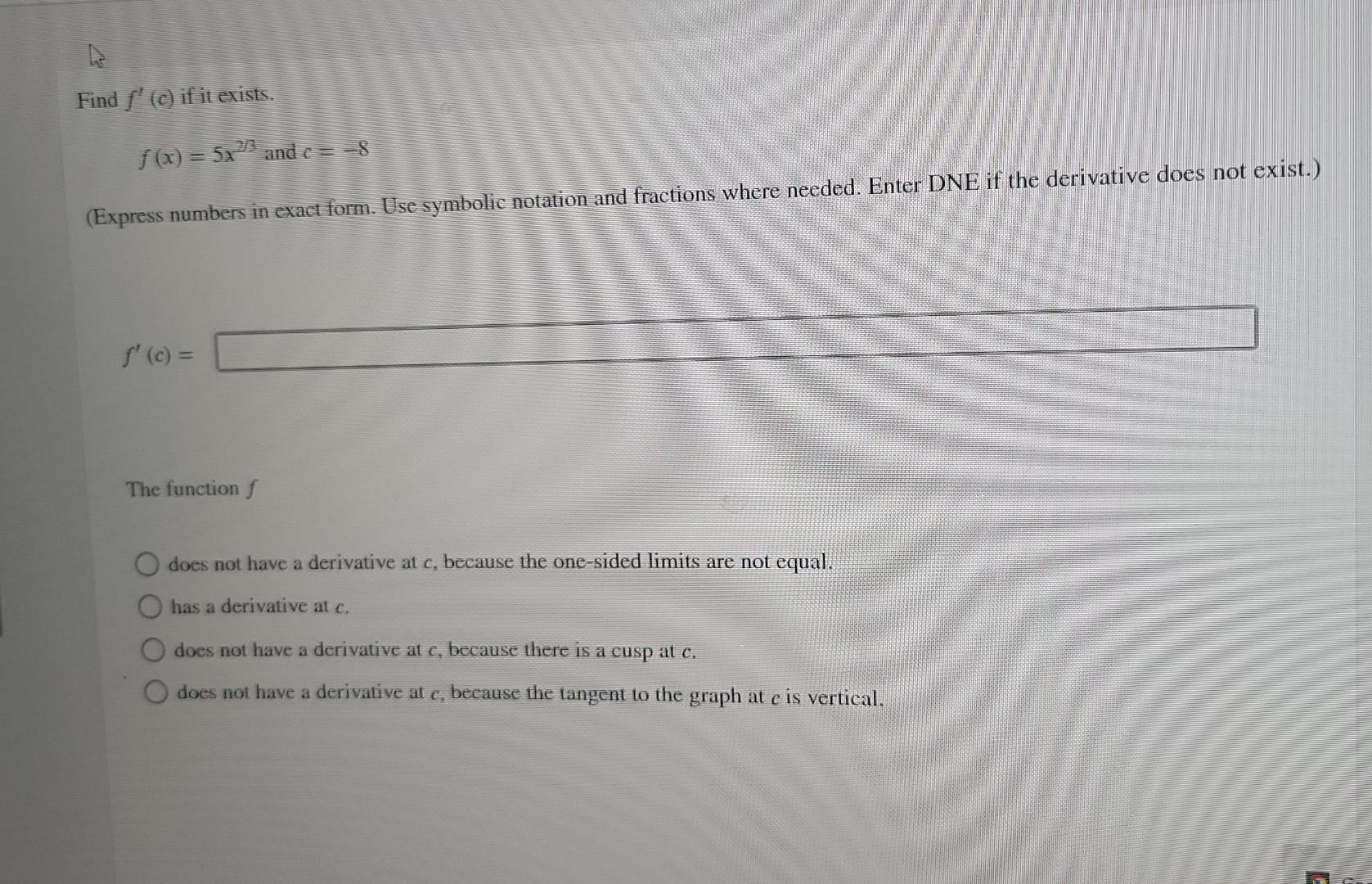# Question Solved1 Answer Find ƒ' (c) if it exists. ƒ (x) = 5x²³³ and c = −8 (Express numbers in exact form. Use symbolic notation and fractions where needed. Enter DNE if the derivative does not exist.) f' (c) = The function f does not have a derivative at c, because the one-sided limits are not equal. has a derivative at c. does not have a derivative at c, because there is a cusp Find ƒ' (c) if it exists. ƒ (x) = 5x²³³ and c = −8 (Express numbers in exact form. Use symbolic notation and fractions where needed. Enter DNE if the derivative does not exist.) f' (c) = The function f does not have a derivative at c, because the one-sided limits are not equal. has a derivative at c. does not have a derivative at c, because there is a cusp at c. does not have a derivative at c, because the tangent to the graph at e is vertical.Transcribed Image Text: Find ƒ' (c) if it exists. ƒ (x) = 5x²³³ and c = −8 (Express numbers in exact form. Use symbolic notation and fractions where needed. Enter DNE if the derivative does not exist.) f' (c) = The function f does not have a derivative at c, because the one-sided limits are not equal. has a derivative at c. does not have a derivative at c, because there is a cusp at c. does not have a derivative at c, because the tangent to the graph at e is vertical.
More
Transcribed Image Text: Find ƒ' (c) if it exists. ƒ (x) = 5x²³³ and c = −8 (Express numbers in exact form. Use symbolic notation and fractions where needed. Enter DNE if the derivative does not exist.) f' (c) = The function f does not have a derivative at c, because the one-sided limits are not equal. has a derivative at c. does not have a derivative at c, because there is a cusp at c. does not have a derivative at c, because the tangent to the graph at e is vertical.